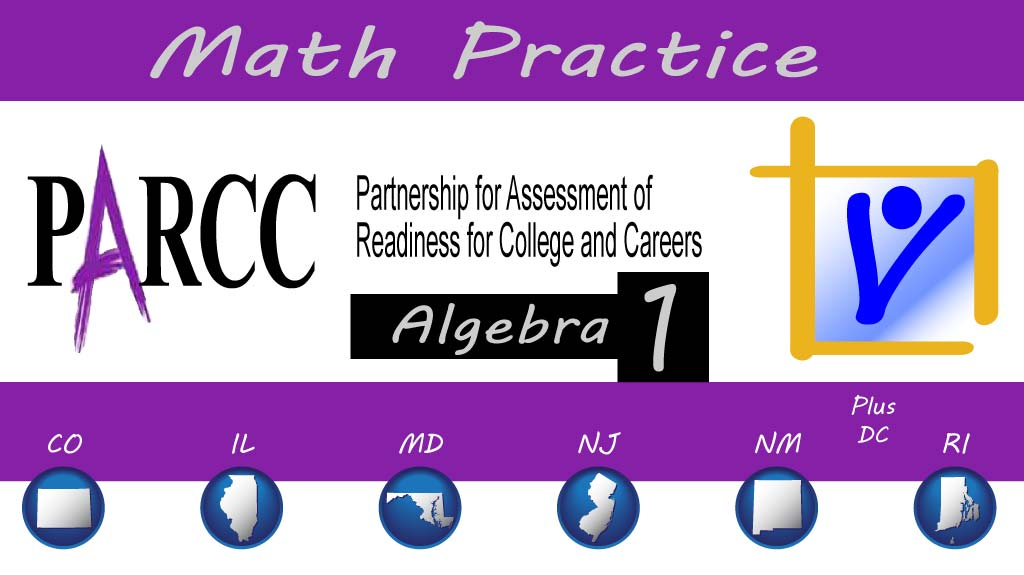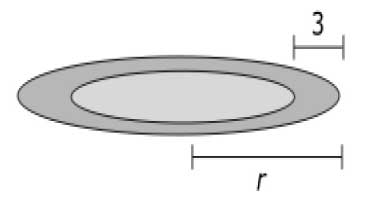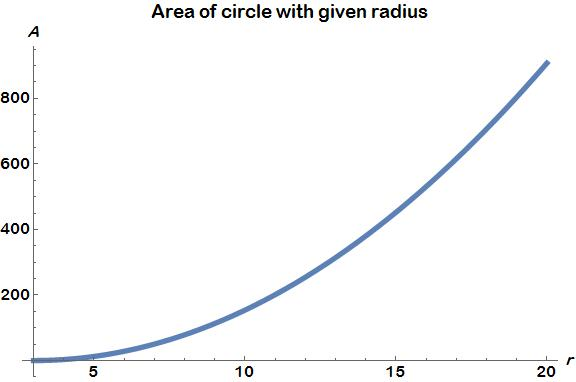Thursday, October 22, 2020# Algebra 1 PARCC: solve literal equation

-

#### The following multiple-choice question, explained here in hopes of helping algebra 1 students and their parents in Maryland and Illinois prepare for the PARCC test near the end of this school year, appears on the released version of PARCC’s Spring 2016 test for algebra 1 (#1):A circular pool of water is shrinking as it drains. The diagram shows the shrinkage.A formula for the area, A, of the circular pool is given by the equation A = π(r – 3)2.

Which is a formula for r?

A.$r = \sqrt{\frac{A}{\pi}}-3$

B.$r = \frac{\sqrt{A}}{\pi}+3$

C.$r = \sqrt{\frac{A}{\pi}}+3$

D.$r = \sqrt{\frac{A}{\pi}-3}$

Correct answer: C:$r = \sqrt{\frac{A}{\pi}}+3$

Common Core Math Content High School, Algebra, Creating Equations:

The question tests students’ understanding of Common Core standards in high school algebra, specifically those under creating equations, which require students to be able to (CED.A) “create equations that describe numbers or relationships” by (CED.A.4) “rearranging formulas to highlight a quantity of interest, using the same reasoning as in solving equations. For example, rearrange Ohm’s law V = IR to highlight resistance, R.”

In this problem, students rearrange the formula for the area of a circle, which is given to them in terms of the radius of the circle, and they are required to rearrange that formula to find the radius of the circle. The formula is a little more complex because of the quantity in parentheses, but the standard is perfectly and validly tested all the same.

Solution strategy (there are others)

Solve the area formula for r.

Starting with the formula given for the area, A, rearrange it with algebra to solve for r:$A = \pi (r - 3)^2$$\frac{A}{\pi} = (r - 3)^2$$\sqrt{\frac{A}{\pi}} = r - 3$$\sqrt{\frac{A}{\pi}}+3 = r$

The area of the circular pool shown in the problem can be graphed with the value of r on the independent axis, as shown here:Note: Values for r are used, not the radius of the pool.

## Analysis of this question and online accessibility

The question is valid in that it tests students’ ability to rearrange equations for the quantity of interest. It measures the Common Core standard it purports to measure. The answer options are all plausible: any of the three incorrect answers can be obtained by making a mistake that is reasonably common as students in algebra 1 classes solve these types of problems.

However, the multiple-choice format does not give an option for partial credit, so there is an all-or-none quality to the question that must be dealt with by providing additional problems on the student’s test for this particular standard. It’s possible that this will lengthen the test in a way that would not be necessary in a non-multiple-choice presentation, where partial credit could be given for completing some of the work.

And the different mistakes students can make aren’t all represented by the wrong answers. If a student makes one of the mistakes that isn’t represented, he’ll know he made a mistake and try again. This is neither bad nor good in terms of the validity of the assessment, but it can lead to frustration if the student is unable to determine what mistake he made.

Guessing isn’t likely on this problem, since the answer options can’t simply be plugged back into an equation and the correct answer isn’t easy to guess through simple observation.

Multiple-choice questions can be delivered online or on paper, and reliability, validity, and fairness performance should be equivalent, regardless of the delivery mode.

No special accommodation challenges can be identified with this question, so the question is considered fair.

(A minor editorial concern with the wording of the question is that the word “which,” used as a pronoun rather than an adjective, does not translate well for some English learners. It is likely that students don’t really read the question carefully anyway and just pick the right answer, which they can figure out without actually reading the question, but sentence structure like this should be avoided in large-scale assessments.)

A not-so-minor editorial concern is that the diagram shows an elliptical pool, not a circular one. The diagram should have been eliminated during the development of this question, since it is irrelevant and doesn’t show what the question says it shows.

## Resources for further study

Purple Math, developed by Elizabeth Stapel, a math teacher from the St Louis area, has a few web pages that demonstrate how to solve literal equations. Her lessons begin here.

The Khan Academy, developed by Sal Khan, an engineer who has created a library of thousands of video lessons, has a series of videos that show students how to solve an equation given with variables on both sides for one of the variables. The series begins here.

Ron Larson, Laurie Boswell, Timothy D Kanold, Lee Stiff. Algebra 2, Illinois edition. Evanston, Ill.: McDougal Littell, a division of Houghton Mifflin Company, 2008. The book is used in several algebra classes taught in Illinois high schools.

Foerster, Paul A. Algebra and Trigonometry: Functions and Applications, revised edition. Addison-Wesley, 1980, 1984. The book is used in several algebra classes taught in middle and high schools in both Illinois and Maryland.

## Purpose of this series

To help algebra 1 students and their parents prepare for the PARCC test in math, as administered in at least six states, or to just master content on that test, we provide an analysis of every algebra 1 math problem PARCC released in 2016. The series can be found here.Paul Katulahttp://news.schoolsdo.org
Paul Katula is the executive editor of the Voxitatis Research Foundation, which publishes this blog. For more information, see the About page.

### Some Chicago-area schools re-close

0
Back-and-forth between e-learning and in-person instruction is caused by the unpredictable nature of the Covid-19 virus.